### Current Trends in Physics

short communication

# Complex Field Theory and Gravity’s New Perspective

### Hossin Abdeldayem*

NASA-Goddard Space Flight Center, Greenbelt, Maryland, USA

*Corresponding author: Hossin Abdeldayem, NASA-Goddard Space Flight Center, Greenbelt, Maryland, 20771, USA

Received Date: 09 August, 2022

Accepted Date: 15 September, 2022

Published Date: 23 September, 2022

Citation: Abdeldayem H (2022) Complex Field Theory and Gravity’s New Perspective. Curr Trends Phys 1: 101 DOI: https://doi.org/10.29011/CTP-101.100001

#### Abstract

Dark matter (DM) and dark energy (DE), despite their abundance, have remained undetected for almost five decades. At the same time, the gravitational force has remained ambiguous since 1687. The complex field theory uncovers their mysteries, reveals their physical nature, identifies the correlation among them, and demonstrates their roles in holding and expanding the universe. The theory’s elegance has been demonstrated through its simplicity in reproducing all general relativity (GR) results without the need for the sophisticated GR equations and space curvature. The theory proposes that DM and DE are complexcharged substances, which carry complex positive and negative dark charges (DCs), respectively. These charged complex substances are also essential ingredients of every material object in the universe. The complex fields (CF), induced by these complex charges of neighboring material objects emulate the gravitational fields and reveals the nature of the gravitational field as a complex field. This new perspective for the gravitational field paves the way for introducing gravity into the standard model.

Keywords: Gravity; General relativity; Dark matter; Dark energy; Dark charge; Complex field; Spacetime curvature; Universe expansion

#### Introduction

Gravity, the most familiar natural force, has baffled the greatest physicists for decades and is still the most intriguing and ambiguous force. This study offers a novel perspective for gravity that proved very useful to incorporate it into the standard model for the first time. This theory has the potential to resolve many other conundrums in physics to be published elsewhere. Since Newton discovered his law of gravity in 1687, scientists have been unable to understand the true physical nature of gravitational fields. At the same time, dark matter (DM) and dark energy (DE), despite their great abundance of ~95% of the universe’s energy, have not yet been detected and their detection remains a challenge. In 1937, Fritz Zwicky was the first to infer the existence of unseen matter, now referred to as dark matter (DM). In the 1980s and early 1990s [1-3], astronomers, and cosmologists became convinced that there was dark energy (DE) holding the whole universe. It was confirmed in the late 1990s [4,5] that the universe’s accelerating expansion could be attributed to a mysterious dark force caused by DM and DE. Our theory uncovers the mysteries surrounding DM, DE, and gravity and their roles of holding and expanding the universe. The theory postulates that both DM and DE are complex charged substances with complex positive and negative dark charges (DCs), respectively. In addition, DM, and DE fill the universe and are essential components of every subatomic particle, in proportion to its mass. The complex field (CF) induced by these DCs emulates the gravitational field and reveals its physical nature. The complex nature of DM, DE, and gravitational field explains the difficulty of directly detecting any of them. The theory elegantly uses the simple Coulomb force and a few simple physics laws to reproduce all results of the general relativity (GR) theory. This new concept of gravity in terms of CFs provides a new prospect for the graviton as a quantization of CF energy and demonstrates it to permeate matter unattenuated and exists between objects and form a threedimensional virtual web connecting the universe.

Theory

The theory is an alternative way of expressing gravity in terms of complex electromagnetic field rather than the real electromagnetic field, which were thought of decades back. The theory postulates the following:

1. DE and DM are substances of complex masses “im”. DM carries positive DCs “iq” and DE carries negative DCs “-iq”. Positive and negative charges balance each other.
2. DM and DE are essential components of each subatomic particle in proportion to its mass, such that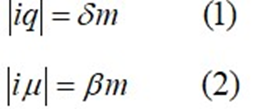Where “d” and “β” are proportionality factors and “q” and “µ” are real quantities. DCs induce complex electric fields (CFs) between every two neighboring objects.

1. Because of the complex nature of DCs, similar charges attract (iq*iq) and opposite charges repel (iq*-iq).
2. Neutral and positive particles carry complex positive DCs, whereas negatively charged particles carry negative DCs.
3. Because nuclei are the most massive components of atoms, all atoms and neutral objects have excess complex positive charges.
4. The DM and DE fill the universe unevenly. DM is highly condensed in massive objects such as atomic nuclei, black holes, neutron stars, and centers of galaxies, and less dense everywhere else, while DE occupies open spaces between galaxies, as will be demonstrated below.
5. The dynamic complex charges on fast objects induce complex electric and magnetic fields, as well as complex scalar and vector potentials, and satisfy Maxwell’s equations.
6. As neutral particles carry positive complex charges, photons carry complex masses (im) and infinitesimal positive complex charges (iq) in proportion to their momenta (h/l).

#### Results and Discussions

Coulomb Force and Gravity

Einstein’s equations of general relativity (GR) have been tested and proven for decades. Our theory does not invalidate or deny its fidelity but offers an alternative complex electromagnetic representation of gravity using the concept of DCs and the use of the much simpler Coulomb’s law. The idea of using electromagnetism to emulate gravity was proposed decades ago after the electromagnetic energy-momentum tensor was implemented in GR theory, but the idea failed to proceed because neutral objects do not interact with electromagnetic fields. The complex field theory resolved the problem by proposing instead the concept of complex electromagnetic field. To do so, the theory postulates that DM and DE are charged complex substances and are essential ingredients of every subatomic material particle. The complex field between objects is a result of these DCs and emulates the gravitational field. Through these postulates, gravity was found to relate to both DM and DE and all three complement each other and control the universe and its expansion.

Newton’s gravitational equation indicates that a small mass “m” is gravitationally attracted to a larger mass “M” by the force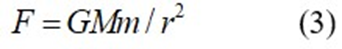Where G is the gravitational constant and “r” is the distance between masses. Einstein’s theory of GR did not formulate a force formula for gravity, considered it a fictitious force, and attributed the attraction between masses to the spacetime curvature caused by the larger mass. However, our theory attributes the attraction to Coulomb force by the DCs carried by the two masses. This prospect presents the gravitational force as a real attractive Coulomb force between the DCs, and the gravitational field between them is the induced CF. This simple attractive Coulomb force was found to mimic the sophisticated GR equations results. The theory couples the Coulomb force and Newton’s gravitational force, as follows:

Coulomb force example between Earth and Moon is: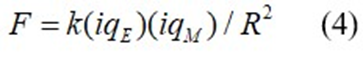where Earth’s mass (ME) carries a charge |iqE|=dME, Moon’s mass (MM) carries the charge |iqM | = dMM, and (k) is Coulomb’s constant. The electric force in (4) is an attractive real force. The field between them is a complex electric field “iE” of real energy density (|iE|2). Equating equation (4) to Newton’s gravitational force in equation (3) and using equation (1), we obtain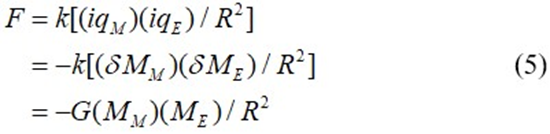The proportionality factor d = 8.611413x10-11 C.kg-1, where d=(G/k)1/2 allows for the quantization of the absolute DCs on Earth |iqE | = 5.143x1014 C, and on the Moon |iqM|= 6.326x1012 C. The complex field “iE” between the two masses exhibits the reason for not being able to detect it directly, while its energy and force are real values and can be directly measured, as was proposed by the “Laser Interferometer Space Antenna (LISA)” to sense the real energy of the gravitational waves. As mentioned, GR does not give an explicit expression for the relativistic gravitational force, but an expression that resembles Newton’s force law, was derived  for the relativistic force as follows: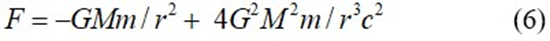The second term accounts for the precession of the elliptical orbits and explains Mercury’s perihelion. The corresponding modified Coulomb expression for equation (6) is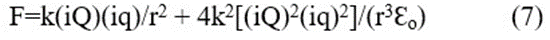Where (iq) is the complex charge on the smaller “m” and (iQ) is that on the larger “M” and Ɛo is the rest mass energy for the smaller mass. Using the coupling constant, “d”, then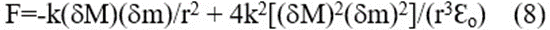Equation (8) allows the estimation of the attractive Coulomb force between relatively large masses, such as black holes on nearby masses. Several expressions for the relativistic gravitational force have been derived [7,8,9] by adding more terms to Equation (6). The corresponding Coulomb force for each can be expressed in terms of their masses by following the same argument, demonstrated above in Equations (7) and (8).

DM, DE, and The Universe

The general theory of relativity did not predict or even discussed DM. Our theory uncovers the correlation of both DM and DE with gravity, and their roles in controlling the universe. As mentioned earlier, the charged DM and DE are essential components of every particle. The center point of each galaxy is a supermassive black hole with 106–1010 times the solar mass. As mentioned earlier, all stars and planets in a galaxy have excessive positive complex charges. The highly condensed positive DM associated with the center mass of a galaxy induces a profound central attractive force and an enormous attractive complex electric field on all stars in its field. The central force induces an orbital circular motion. This means that the DM, associated with the central Black Hole (BH), is the source of the force holding and causing the orbital circular motion of a galaxy. Simultaneously, the counterclockwise circular motion of positively charged matter in a galaxy induces a complex magnetic field perpendicular to the plane of the circulation. This complex magnetic field induces a centripetal force on the positive matter (DM) towards the center, contributing more to the holding of the galaxy and a centrifugal force on the negative complex charges (DE) and ejects them out of the galaxy to fill in the space between galaxies. This negatively charged DE in the space between galaxies induces a positive repulsive force [(iq)(−iq)] on the positively charged matter of the galaxy. Consequently, DE behaves as a buffer zone separating galaxies, and its repulsive force on the galaxies contributes to the expansion of the universe. This repulsive effect of DE was hypothesized by Einstein in 1917 and made him introduce the cosmological constant in his equations to account for the universe’s expansion. This repulsive force between DE and DM can also be examined from a thermodynamic point of view, as follows:

The first law of thermodynamics states that energy cannot be generated or destroyed. The energy density “Too” of the complex electromagnetic stress-energy tensor in each galaxy is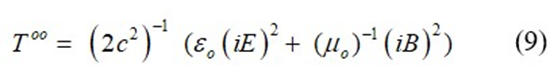Where iE and iB are the complex electric and magnetic fields, respectively. This negative stress-energy density must be accompanied by positive pressure to maintain the total energy of the universe constant. This positive pressure is another expression for the repulsive force mentioned above, which contributes to the universe’s expansion. In conclusion, DE and DM are the engines that hold the galaxies and expand the universe [10,11].

The Lensing Effect of the Sun’s Atmosphere

The historic solar eclipse experiment on May 29, 1919, demonstrated the gravitational lensing effect of the sun and confirmed the spacetime curvature concept of the GR. The complex field theory explains the lensing effect as a third-order nonlinear optical effect, induced by the DCs and their CF. Nonlinear optical effects occur when an external electric field acting on a nonlinear medium approach or exceed the medium’s atomic fields of 107–1010 V/cm. Massive stars carry extensive complex positive charges, which induce a substantial complex electric field “iE” and a profound electric field intensity near its surface. For example, in the Sun’s case of mass ~ 2x 1030 kg and a dark charge |iq| = 1.7x1020 C, the induced field intensity on its atmosphere is several orders of magnitude greater than the atomic field in its atmosphere. This excessive field intensity varies with the height from the surface of the sun, and accordingly, gradient modulation of the refractive index of the atmosphere is induced at different heights. It is maximum near the surface and reduces away from the surface. The refractive index of matter, in general, results from the induced polarization of electrons in the atmosphere by the sun’s complex electric field. The polarization formula is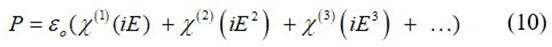The first term is complex and induces no polarization effect on the electrons in the atmosphere, and consequently causes no modulation to the refractive index. This implies that the complex field permeates unattenuated through the atmosphere. In other words, matter is transparent to the complex field. The second term in Equation (10) has a real value and is a measure of the field intensity. Nonlinear polarization due to this term occurs only in certain types of crystals, which lack inversion symmetry and cause second-order nonlinearity and second-order frequency conversion. They do not affect liquids, gases, or centrosymmetric crystals. This means that it does not affect or interact with the Sun’s gassy atmosphere. The third term in equation (10) is the most common term in conventional optics and is responsible for third-order nonlinearities and third-order frequency conversions. They play a major role in gases, plasma, liquids, and centrosymmetric or isotropic crystals. The second- and third-order optical nonlinearities have been studied extensively in many textbooks [12,13,14]. The complex field in the third term in Equation (10) interacts with the complex refractive index of the absorptive atmosphere and causes nonlinear refractive index modulation. This term modulates the refractive index in the sun’s atmosphere as: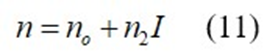Where “n2I” is the intensity-dependent modulation to the refractive index “no”. The extremely hot sun’s atmosphere induces an excellent nonlinear medium for several nonlinear mechanisms to take places such as thermal nonlinear mechanism, Kerr effect, two-photon absorption, plasma nonlinearity, etc. Although the thermal nonlinear mechanism is the most dominant, each of the others will also play a role and cause refractive index modulation at different levels. Each mechanism induces a nonlinear prism  and each prism deflects the light beam from a straight line, depending on its strength. The beam deflection by all prisms perfectly mimics the results of the 1919 lensing experiment.

Slowing Down of Time near a Black Hole

In general, a light beam does not feel an electric field and travels straight and unaffected through it. Our theory offers a different account for the slowdown of time near a black hole. A massless neutral photon carries an infinitesimal positive complex charge that interacts while traveling through the intense complex field of the BH. Black holes are massive objects that range between the stellar class of masses of three to ten times the solar masses and the supermassive ones of masses that are up to billions of solar masses. A supermassive BH contains extremely dense DM along with an extraordinarily concentration of complex positive charges, and a tremendous complex electric field “iE”. Each infinitesimally complex charged photon passing through this intense complex electric field reacts to it as the electron interacts with an electric field , except that the Hamiltonian for the photon in our case can be expressed as:

of “m”. Note that the gravitational field between the Moon and the Earth is different in magnitude from that between the Earth and the Sun, depending on the DM and the DCs on the center mass. Expressing gravity in terms of a complex electric field facilitates incorporating gravity into the standard model, where the gravitational Lagrangian density can be expressed as: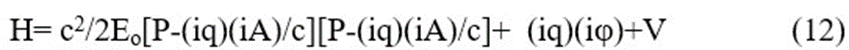where Eo is the photon energy, P-its momentum operator, (iq) is its complex charge, (iA) is its vector potential, (ij) is its scalar potential, and V=(iqP)(iQBH)/r is the negative extraneous potential energy of the BH on the photon charge. Each photon will experience a force: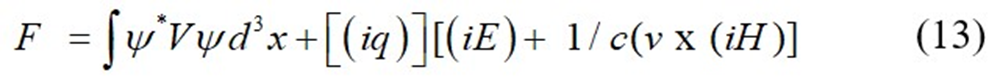This is equivalent to the classical force of an electromagnetic field acting on an electron. The force in Equation (13) is negative (attractive) and has these two meanings.

1.       It represents an attractive force between the infinitesimal small positive complex charge on the photon and the extremely dense complex field of the BH. This attractive force tends to bend the beam from its straight path towards the BH. If the beam is too close below a threshold height from the surface of the BH, it will end up falling into the BH and will never be seen.

2.       Above the threshold height from the BH surface, it is deflected towards the surface from a straight line, this negative force acts as a decelerating force, and decelerates the speed of the photon to a slower speed than its speed in a vacuum and causes the beam to slow down. Note that the electric field of the BH at r=0 is infinite, the retarding force also becomes infinite, and the photon will never escape the BH.

Gravity and the Standard Model

Gravity is the most obscure among the four forces of nature. Because its field is ambiguous, GR considers it a fictitious force. The gravitational acceleration from equation (3) for a mass “m” is g=GM/r2, which is independent of “m”. The attractive Coulomb force of the mass “m” of complex charge (iq)/d, is real [F=k(iQ)(iq)/r2], and its acceleration [a=(d/i)[k(iQ)/r2]] is also real and independent

In general, a light beam does not feel an electric field and travels straight and unaffected through it. Our theory offers a different account for the slowdown of time near a black hole. A massless neutral photon carries an infinitesimal positive complex charge that interacts while traveling through the intense complex field of the BH. Black holes are massive objects that range between the stellar class of masses of three to ten times the solar masses and the supermassive ones of masses that are up to billions of solar masses. A supermassive BH contains extremely dense DM along with an extraordinarily concentration of complex positive charges, and a tremendous complex electric field “iE”. Each infinitesimally complex charged photon passing through this intense complex electric field reacts to it as the electron interacts with an electric field , except that the Hamiltonian for the photon in our case can be expressed as:

of “m”. Note that the gravitational field between the Moon and the Earth is different in magnitude from that between the Earth and the Sun, depending on the DM and the DCs on the center mass. Expressing gravity in terms of a complex electric field facilitates incorporating gravity into the standard model, where the gravitational Lagrangian density can be expressed as:

L = ½ (∂(iE))2 − ½ µ2(iE)2                                     (14)

Where E is the real value of the complex field and “m” is the real value of the DM on the heavier mass. Equation (14) can be expressed in terms of the center mass “M” as:

L =1/ 2 (∂(iE))2 – ½ (βM)2(iE)2                           (15)

Which is the Lagrangian density expression for a gravitational mass in the standard model.

#### Conclusion

This theory resolves and uncovers several old conundrums in physics. First, the gravitational field was considered ambiguous since Newton discovered his gravitational law in 1687. This theory unveils its ambiguity and defines it as a complex electric field. Second, the theory of GR introduces the spacetime curvature concept to explain gravity. This theory demonstrates that GR can be calculated without spacetime curvature. Third, the theory reproduces all the gravitational phenomena of GR such as the lensing effect, the slowing of time near a black hole, and the universe’s expansion. Fourth, the theory paves the way for gravity to be incorporated into the standard model. Fifth, the concept of complex charges as essential ingredients of every subatomic particle grants life to neutral particles and inanimate objects, which clears Newton’s confusion about the spooky action at a distance between two inanimate masses without anything in between. Sixth, the model demonstrates that DM and DE are the driving engines for the universe and the cause for its expansion. This new perspective for DM and DE in the universe replaces the old conviction which says, “mass tells space how to curve and space curvature tells mass how to move.”

Acknowledgments

The author thanks Dr. Amidu Oloso, Erin Majerowicz, and Samantha Kilgore for their valuable feedback and editing the manuscript. The author has declared that no competing interests exist. The study was approved by the Ethics Office of the General Counsel at NASA-Goddard Space Flight Center. The paper is a pure theoretical work, and no external funds were needed to support, publish, or prepare the manuscript. All relevant data are within the paper.

#### References

Copyright and Licensing: This is an Open Access Journal Article Published Under Attribution-Share Alike CC BY-SA: Creative Commons Attribution-Share Alike 4.0 International License. With this license readers can share, distribute, download, even commercially, as long as the original source is properly cited. Read More.